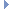OpenMM
Discrete1DFunction Class Reference

This is a TabulatedFunction that computes a discrete one dimensional function f(x). More...Inheritance diagram for Discrete1DFunction:

List of all members.

## Public Member Functions

def __init__
__init__(self, values) -> Discrete1DFunction
def getFunctionParameters
def setFunctionParameters
def Copy
Copy(self) -> Discrete1DFunction.

this

## Detailed Description

This is a TabulatedFunction that computes a discrete one dimensional function f(x).

To evaluate it, x is rounded to the nearest integer and the table element with that index is returned. If the index is outside the range [0, size), the result is undefined.

## Constructor & Destructor Documentation

 def __init__ ( self, values )

__init__(self, values) -> Discrete1DFunction

This is a TabulatedFunction that computes a discrete one dimensional function f(x). To evaluate it, x is rounded to the nearest integer and the table element with that index is returned. If the index is outside the range [0, size), the result is undefined.

## Member Function Documentation

 def Copy ( self )

Copy(self) -> Discrete1DFunction.

Reimplemented from TabulatedFunction.

 def getFunctionParameters ( self )
 def setFunctionParameters ( self, values )

## Member Data Documentation

The documentation for this class was generated from the following file:
• openmm.py